Courses

# Network Synthesis - 2

## 15 Questions MCQ Test Topicwise Question Bank for Electrical Engineering | Network Synthesis - 2

Description
This mock test of Network Synthesis - 2 for Electrical Engineering (EE) helps you for every Electrical Engineering (EE) entrance exam. This contains 15 Multiple Choice Questions for Electrical Engineering (EE) Network Synthesis - 2 (mcq) to study with solutions a complete question bank. The solved questions answers in this Network Synthesis - 2 quiz give you a good mix of easy questions and tough questions. Electrical Engineering (EE) students definitely take this Network Synthesis - 2 exercise for a better result in the exam. You can find other Network Synthesis - 2 extra questions, long questions & short questions for Electrical Engineering (EE) on EduRev as well by searching above.
QUESTION: 1

### Which of the following is not a Hurwitz polynomial?​

Solution:

For option (a):
F1(s) - (s + 1) (s2 + 2s + 3)
= s3 + 3s2 + 5s + 3
Since all coefficients of F1(s) are positive and no term is missing, therefore it is a Hurwitz polynomial.
Now, F2(s) - (s + 3) (s2 + s - 2)
= s3 + 4s2 + s - 6
Since F2(s) has a negative coefficient, therefore
it is not a Hurwitz polynomial
Similarly, we can check for options (c) and (d).

QUESTION: 2

### A Hurwitz polynomial has

Solution:

A Hurwitz polynomial must have no poles and zeros in the RH s-plane.

QUESTION: 3

### The function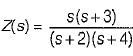represent a

Solution:

The pole-zero plot of Z(s) is shown below.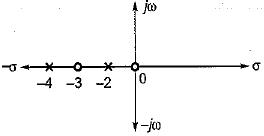Here, singularity nearest to origin is a zero while near infinity is a pole. Also, pole-zero alternates on negative real axis.
Therefore, Z(s) will represent a R-L impedance function.

QUESTION: 4

The circuit shown in the figure is a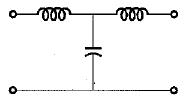Solution:

For s → 0, L acts as short circuit and C acts as open circuit.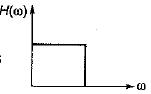Thus, V0 = V
For s → ∞, L acts as
open circuit and C acts as short circuit.
∴ V= 0
Hence, given circuit will act as a LPF.

QUESTION: 5

The driving point admittance of the network shown below is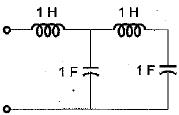Solution:

Converting the given network in Laplace domain, the driving point impedance is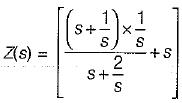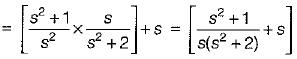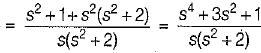∴ Driving point admittance V(s)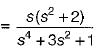QUESTION: 6

Which of the following is a PRF (positive real function)?

Solution: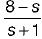is not a PRF since all the coefficients of numerator polynomial are not positive and there is a zero in RH s-plane.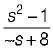is not a PRF due to the same reason (zeros are at s = ± 1).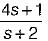is a PRF since pole is at s =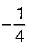and zero at s = - 2 .
Also, all coefficients of N' and Dl are positive.
And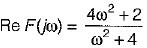and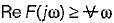QUESTION: 7

A function F(s) is defined as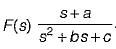The necessary condition required for F(s) to be a positive real function is

Solution:
QUESTION: 8

What is the range of values of m in P(s), so that P(s) is a Hurwitz polynomial?
P(s) = (2s4 + s3 + ms2 + s + 2)

Solution:

Given P(s) - 2s4 + s3 + ms2 + s + 2
= (2s4 + ms2 + 2) + (s3 + s) = M(s) + N(s)
Continued fraction expansion of M(s)/N(s) is obtained as follows: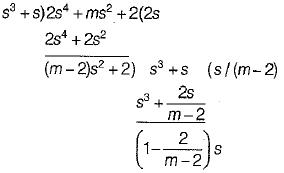The quotients of the continued fraction expansion would be positive only when m > 2 and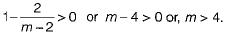Hence for m > 4, P(s) will be Hurwitz.

QUESTION: 9

Consider the impedance functions: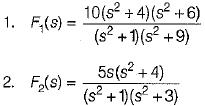Out of the above impedance function which are driving point LC immittances of LC network?

Solution:

The pole-zero plots of F1(s) and F2(s) are shown below.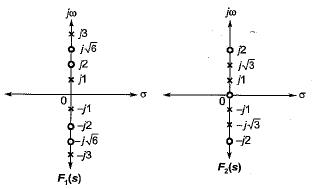In Z1(s): Poles and zeros do not alternate. Also, there is no zero-or a pole at origin.
In Z2(s): Poles and zeros do not alternate. Hence, neither of Z1(s) or Z2(s) represents a LC immittance function.

QUESTION: 10

Driving point impedance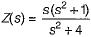is not realizable because the

Solution:
QUESTION: 11

Cauer and Foster forms of realizations are used only for

Solution:
QUESTION: 12

The driving point impedance function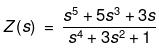can be realized as

Solution:

The continued fraction expansion is shown below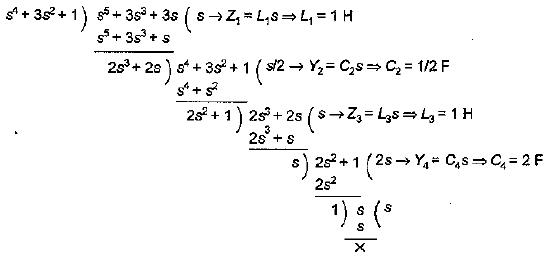Hence, Z(s) can be realized as a L-C network.

QUESTION: 13

Match List - I (Network functions) with List - II (Type of functions) and select the correct answer using the codes given below the lists:
List - I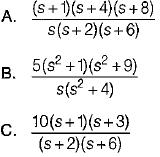List - II
1. LC immittance function
2. RC admittance function
3. RL admittance function
4. RLC network function
Codes: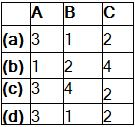Solution:
QUESTION: 14

Assertion (A): Foster’s type - I and Foster’s type - II networks are equivalent networks.
Reason (R): Foster’s type - I and Foster’s type - II networks are dual of each other.

Solution:

Foster’s type - I and type - II networks are not equivalent networks.
Hence, assertion is false.

QUESTION: 15

Assertion (A): The realization of one port LC networks can be done in two. configurations (commonly known as Cauer-I and Cauer-II forms) using the continued fraction expansion of the driving point impedance.
Reason (R): The basic form of the Cauer realization being a ladder type network.

Solution: